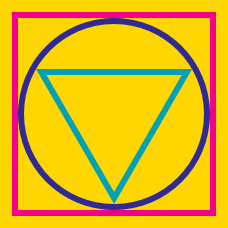Geometry

# Inscribed and Circumscribed Figures: Level 2 Challenges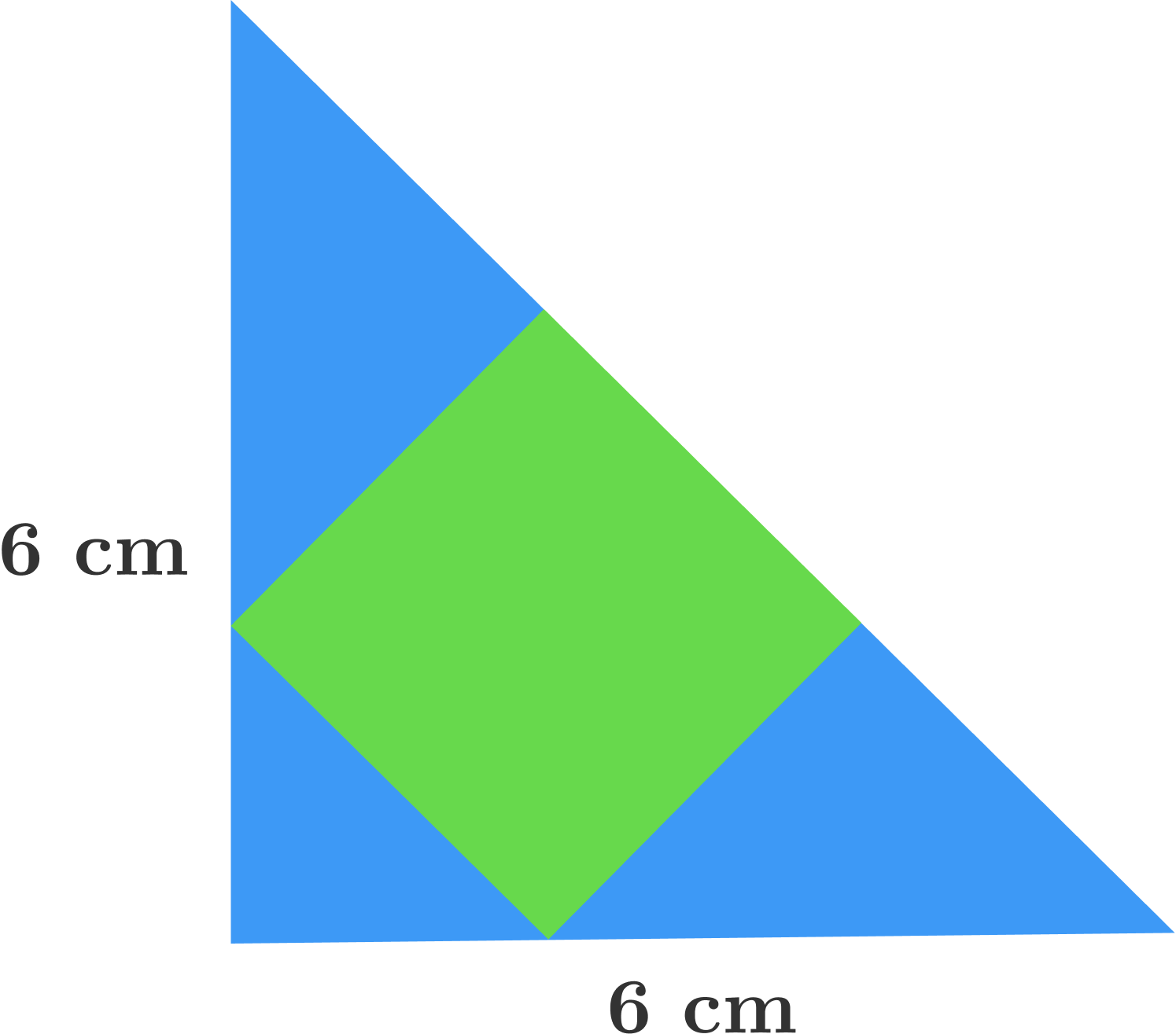Find the area (in $\text{cm}^2$) of the shaded green square in the blue isosceles right triangle, whose legs have length 6 cm.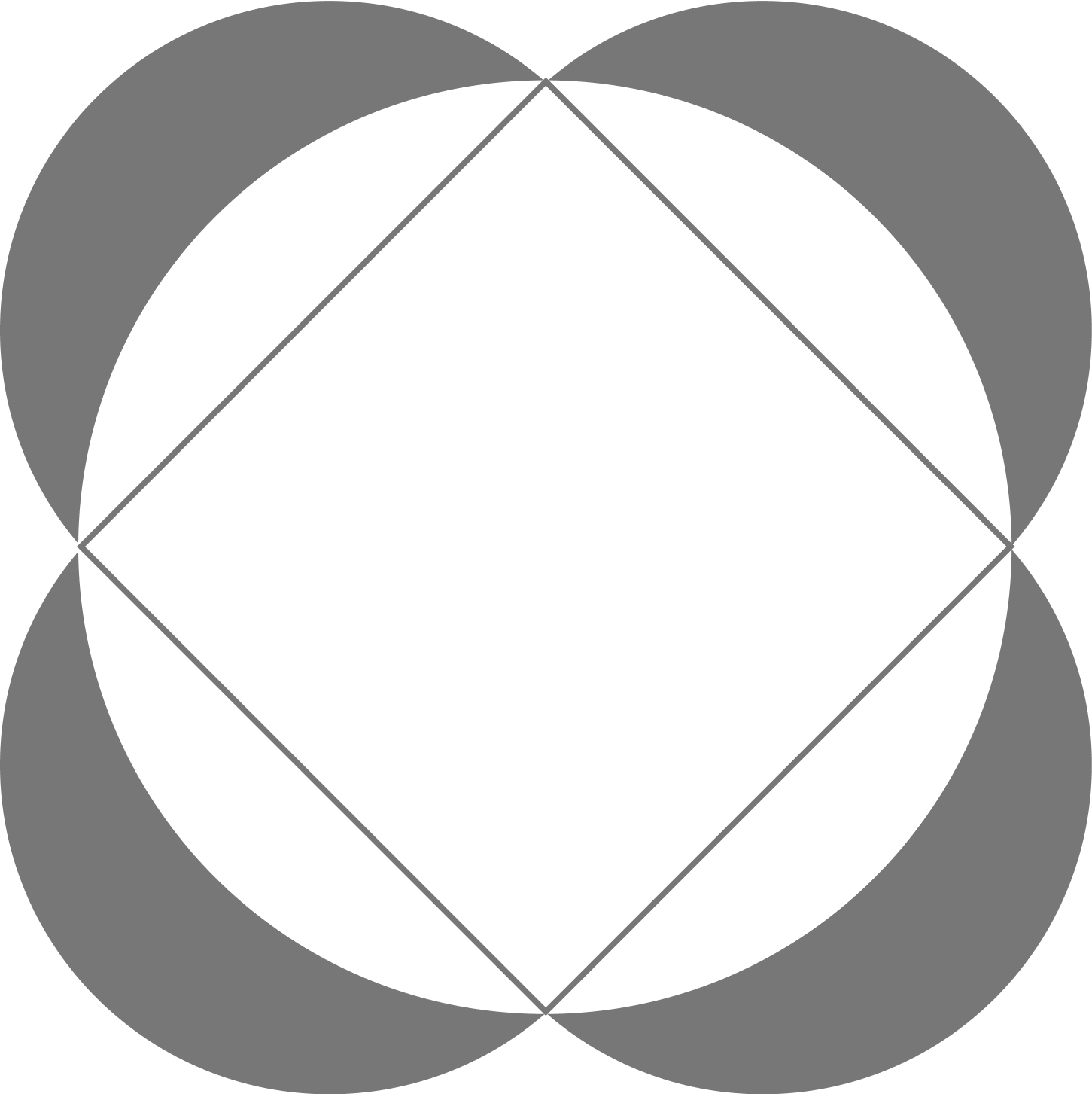A square is inscribed in a circle with diameter 2. Four smaller circles are then constructed with their diameters on each of the sides of the square. Find the shaded area.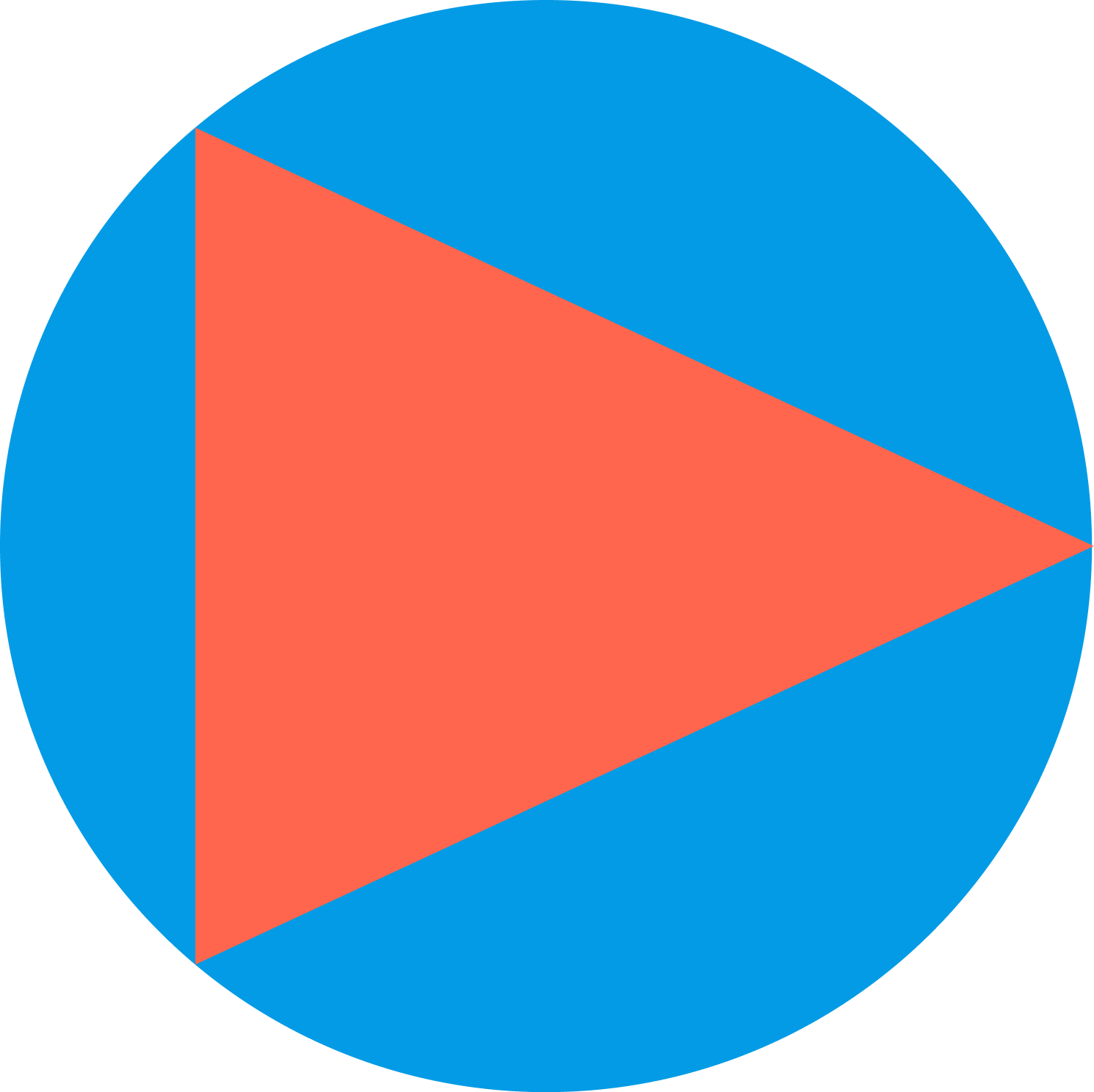In a circle of radius 1, an equilateral triangle is inscribed in the circle as drawn. What is the area of the blue region?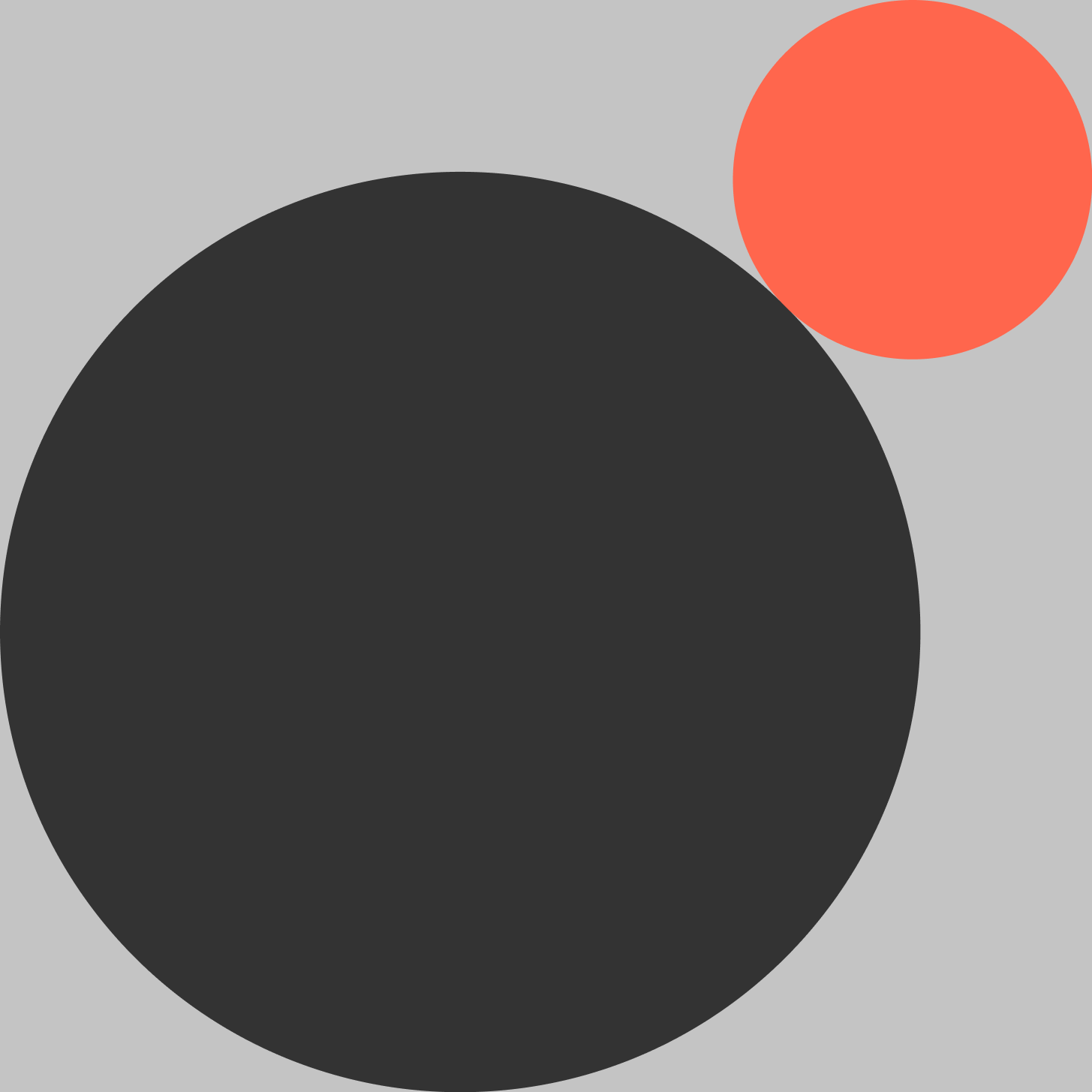Two circles are drawn inside a square with side length $2+\sqrt{2}$ as shown. Let the radius of the larger circle be $R$ and the radius of the smaller circle be $r$. Find the value of: $R + r$There is a quadrant-shaped field partitioned into four sections by the intersection of two semicircles with bases at the perpendicular axes of the quadrant. What is the ratio of areas of the red section to the blue section?

×